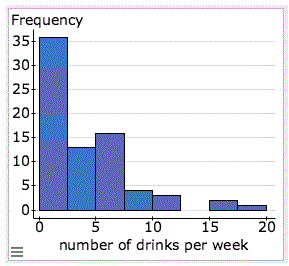# hypothesis test for a population mean lab

### Prompt

The drinks datafile is available in the Data section below.

1. Let$Î¼$

be the mean number of alcoholic beverages that students in the liberal arts college drink in a typical week. State the hypotheses.

2. Here is a histogram of the data. Can we safely use the t-test with this data? Explain.3. Perform the t-test using StatCrunch. (directions)
Copy and paste the information from the StatCrunch output window into your initial post.
4. Hypothesis test results:
5. Variable Sample Mean Std. Err. DF T-Stat P-value
number of drinks per week 3.9333333 0.43592434 74 -1.8275343 0.0717

6. Based on the P-value, state your conclusions in context. Use a 5% level of significance.
NOTE: You do not need to double the P-value for this two-tailed test. StatCrunch calculates the P-value for you.
7. Using the context of this scenario, explain the meaning of each of following items from the StatCrunch output for the hypothesis test.
1. Std. Err (standard error)
2. T-stat (T-score)
3. P-value